In the current world, data is growing progressively, with larger or more complex computation problems needing to be solved. What should we do about solving problems that don’t fit on a single core CPU or can’t be solved in reasonable time?

Parallel programming not only solves these problems but also reduces computation time. All computers are multi-core processors these days, even your iPhone 4s has two cores. In this article, I will look over the possible scenarios where we can apply parallel computing and the factors affecting computation time. We will also look into the benchmarking of parallel programs.

Parallel computing is a type of computation where different computations can be performed at the same time.

Basic principle: The problem can be divided into subproblems, each of which can be solved simultaneously.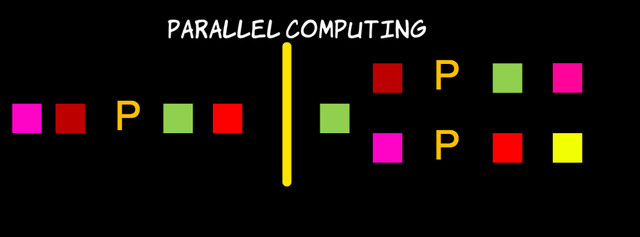Many of us cannot find how parallel programming differs from concurrent programming. Let’s take a step back and understand the concept and reasoning behind parallel computation.

Parallel computation:

• Optimal use of parallel hardware to execute computation quickly
• Division into subproblems
• Mainly used for: Algorithmic problems, numeric computation, Big Data processing

Concurrent programming:

• May or may not offer multiple execution starts at the same time
• Mainly concerns about: Convenience, better responsiveness, maintainability

There are different levels of parallelism e.g. bit level, instruction level, and task level. For the purpose of this article, we will focus on task level parallelism.

Before getting into parallel programming problems, let’s first understand how the hardware behaves.

Processor vendors like IBM and Oracle provide a multi-core CPU on the same processor chip, each of which is capable of executing a separate instruction stream:

• Multi-core processor
• Symmetric multiprocessors
• Graphic processing unit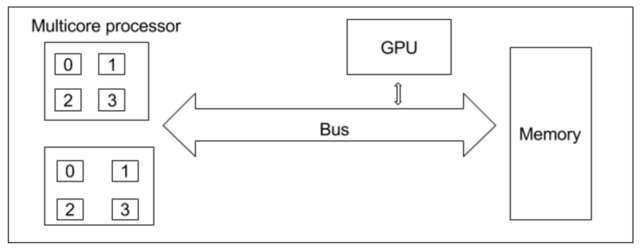Parallel programming is much harder than sequential programming. It even makes developer life harder. However, the speed at which results can be delivered is a big plus on the side of parallel programming.

The operating system and JVM are the underlying environments which make parallelism tasks possible. As we know, two different processes don’t share the memory which serves as a blocker for running the task in parallel. But each process contains multiple independent concurrent units called threads. Threads share the same memory address space.

A task can be stated as executing separate instruction streams in parallel.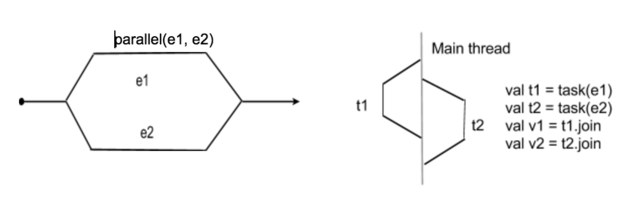The signature of parallel execution, where taskA and taskB are called by name, means it doesn’t execute sequentially.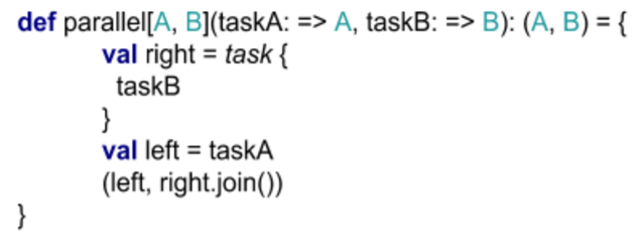Here you can see the method ‘task’, which is scheduled on a separate thread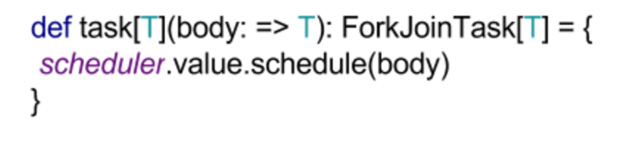Let’s look at an example:

Find the total number of ways a change can be made for the specified list of coins for the specified amount of money.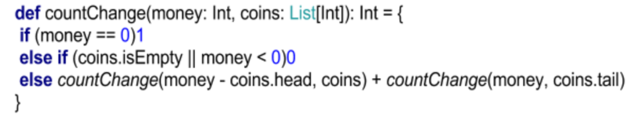This method “countChange” runs sequentially and produces a result in ‘82.568 ms’: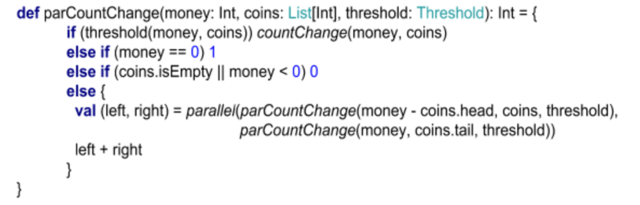Whereas the parallel version runs in ‘48.686 ms’ with a speedup of ‘1.695 ms’.

What hardware are we using for this example? The MacBook Pro "Core i5":

• Processor 2.4 GHz (dual independent processor "cores" on a single silicon chip)
• Memory 8GB

Of course you can’t parallelise everything. Let’s try to understand the cost of splitting and combining the data structures, such as arrays.

Assume we have a four core processor and an array size 100 over, which we have to perform a filter operation on.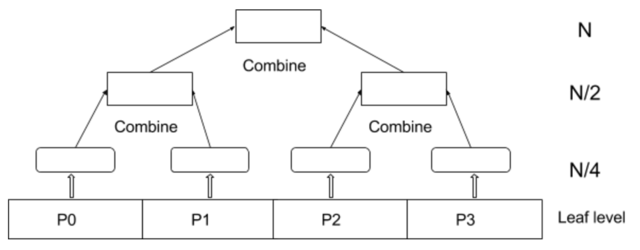At the leaf level of the parallel reduction tree, it will traverse N elements in N/4 computational steps and perform filtering. Process P0 and P1 produces two arrays and, in similar way, P2 and P3 also.

In the next step, two arrays will be combined together in N/2 computational steps. Finally, at the root level, we have to combine two arrays which take N computational steps.

While summing up all the computational steps, we have found that 7N/4 > N > N/4.

The overall complexity is O(n + m), which is not efficient. Also, we can see that combining takes as much time as filtering does. Let’s define the combine operation in order to understand it.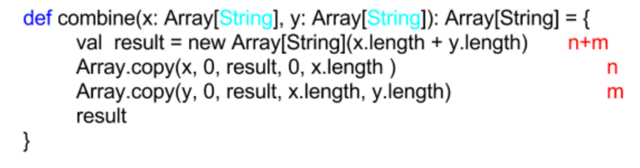As we can see, it will take 2n + 2m = 2(n+m). In complexity analysis constants are ignored, hence O(n+m).

The data structure looks like this:

• HashTables compute the hashcode of the elements where lookup, insert, and delete operations take constant time O(1)
• Balanced tree expect O(logn) to reach any Node
• Mutable linked list can have O(1) concatenation

With this we can see that it’s possible to combine data structures in trivial time. In order to proceed with parallel computation, we have to perform the execution in O(logn + logm) time.

### Why should we benchmark parallel programs?

Performance benefits are the main reason why we write parallel programs in the first place. It computes performance metrics for parts of the program, which is why it’s even more important than benchmarking sequential programs.

Factors affecting performance:

• The number of processors
• Latency while accessing memory and throughput
• Processor speed
• Cache behavior
• Garbage collection
• JIT compilation

ScalaMeter is a benchmarking and performance regression testing framework for the JVM which is a great tool for this purpose. It computes performance metrics for the part of the program. The metrics could be running time, memory footprint, network traffic, disk usage, and latency.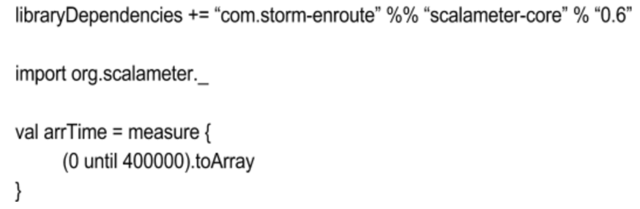### Summary

In this article I have covered the basic principle of parallel programming, the role of hardware resources, complexity, and benchmarking. I would like to highlight a few important points which usually pop up while designing parallel computation algorithms.

• Limit of parallelisation is bound by number of cores
• Use of threshold, even though you have enough cores in order to avoid parallelism overhead.

Then there's the question of when should you NOT use parallelised computation?

• If you do not have enough parallel resources: Whether it is the hardware core and its abstraction though a thread pool, or something less obvious like memory bandwidth.
• If the overhead of parallelisation exceeds the benefit: Even if you have enough cores, there is overhead in doing computation in parallel. This includes, among other things, spawning the tasks and scheduling
• Both of the above mentioned conditions alone can make parallel execution less efficient than sequential ones, so a robust algorithm should ensure that none of these issues come up.

Consider the case when the array has only 100 elements and we have to perform an addition of elements in parallel on several cores. Here the overhead of parallelising the computation is not worth it, irrespective of how many cores you have. It’s simply not enough data.

On the other hand, if we have an array with 1000 elements and we have to perform filtering or any other operation upon it, than parallelising the computation becomes worth it.

I hope to keep exploring this area and am working on coming up with a tutorial or workshop. In the meantime if you have questions, you can reach me through Twitter at @mohdnadeem.